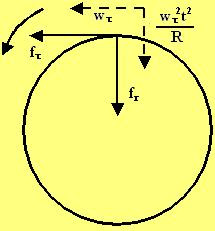## Sunday, October 21, 2007

### Irodov Problem 1.90From the point of view of a stationary observer, as the car rotates at a radius R, he is subject to centripetal acceleration direction radially inwards given byat a time t. Further, because the car is accelerating it has a tangential acceleration of. Both these acceleration components are provided by the components of friction force acting in the two directions(tangential) and(radial) . Thus based on newton's laws,The net force of friction is the Euclidean norm of the two components,But the maximum force of friction that can be offered is mgk thus, at slipping point,The distance traveled by the car will be,